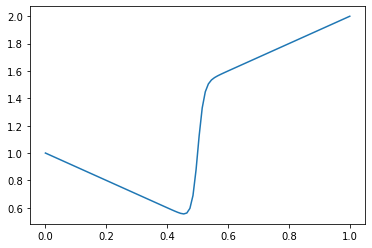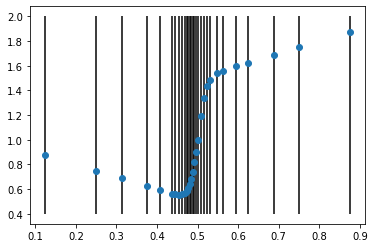Fancy pants¶

Suppose we have a function like the following below.

In :
import numpy as np
import matplotlib.pyplot as plt
%matplotlib inline
In :
def f(x) :
return x * np.tanh(50.0*(x-0.5)) + 1.0

x = np.linspace(0,1,100)
plt.plot(x,f(x));Some parts change slowly (or change uniformly), and some parts change quickly.

Question, how would you set the step size to get an accurate integral using the trapazoid method?

• Answer, use variable width trapazoidsQuestion, how do you know where to put the variable widths?

Question, what if you want adaptive trapazoid widths for any function, even ones you don't know yet?

* That is, for any function that a user might supply.
* How can you make your code decide?

• Put points uniformly along the curve of the function.

All of these are pretty complicated, there is a simpler way

• The simplest possible application of the trapazoid method is to use a single trapazoid.
• call this $I_1$
• The next simplest is to use two trapazoids.
• call this $I_2$
• Now, compare $I_1$ and $I_2$.
• If they are close enough then we are done.
• If they are different, then do the same 1:2 trapazoid comparison in each half of the domain.
• Repeat in each subregion.• This can be done with a recursive algorithm
In :
def adapt_trap(f, a, b, tol) :

m = (a+b)/2  # midpoint

global store_m; store_m += [m]   # store the midpoint for plotting below

I1 = (b-a)*(f(b)+f(a))/2                         # 1 trap
I2 = (m-a)*(f(a)+f(m))/2 + (b-m)*(f(m)+f(b))/2   # 2 traps

if np.abs((I1-I2)/I2) <= tol : # done if I1, I2 are close
return I2
else :                         # recursive: integrate the 2 sub intervals